﻿ Clemson Vehicular Electronics Laboratory: Asymptotic-Expansion Methods# Asymptotic-Expansion Methods

The techniques described in the previous sections are exact methods in that the error in the numerical solution only comes from the discretization. The numerical solution approaches the exact solution as the discretization is refined. However, as the number of unknowns grows, the demand for computer memory and calculation time also grows. This prohibits these methods from being applied to high frequency problems where the size of the object is much larger than the wavelength. The methods described in this section are based on asymptotic high-frequency expansions of Maxwell's equations. They are high frequency methods that are only accurate when the dimensions of the objects being analyzing are large compared to the wavelength of the field. The asymptotic techniques introduced in the following sections include physical optics, geometrical optics, geometrical theory of diffraction, and uniform theory of diffraction.

## Physical Optics

The Physical Optics (PO) approximation is a well known and efficient method for analyzing large scatters . PO reduces the cost of memory and CPU-time by performing a high frequency approximation. It is a current-based method in which the physical optics approximation is used to obtain the current density induced on a surface. The surface current density, Js, can be determined by,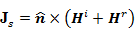(1)

where Hi and Hr represent the incident and reflected magnetic field components evaluated on the surface.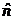is the unit vector normal to the surface. If the surface can be approximated as an infinite plane surface, then by image theory,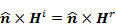(2)

and Equation (1) reduces to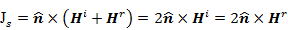(3)

The electric and magnetic field radiated by the surface current on the illuminated side of the reflector can be determined by ,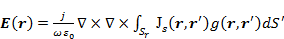(4)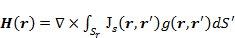(5)

where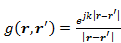.

Equation (3) is exact only when the surface is infinitely large. The accuracy of the approximation depends on the transverse dimensions of the reflecting surface, the radius of curvature, location of edges, and the angle of the incident field. Generally, PO works well for large, smooth surfaces with low curvature. The implicit assumption for the physical optics approximation is that the incident field is treated as a locally planar wave. Also, it assumes that the reflector surface is perfectly conducting.

It has been found that PO provides an accurate prediction of far-field patterns of reflected antennas in the main beam region and out to several side lobes . The major disadvantage of PO is that the integration over the surface of the reflector may be quite complicated and time consuming when the feed is placed off-axis or the feed pattern is asymmetric . Moreover, the radiation integral has to be evaluated each time the observation point is changed.

Fast and efficient evaluation of the radiation integral was proposed using a fast series approach , incorporating a multilevel fast multipole method , or decomposing the scatterer into subdomains . Initially applied in the frequency domain, PO has also been extended into the time domain .

## Geometrical Optics (Ray Optics)

Geometrical Optics (GO)  or geometrical optics with aperture integration (GO/AI) is a ray-based method intended for the consideration of electrically large dielectric structures in applications like the analysis of reflector antennas. In GO analysis, geometrical optics techniques (ray tracing) are used to set up equivalent currents on an aperture plane which is normal to the axis of the reflector. Then, the tangential aperture fields are constructed and used to determine the radiated fields utilizing the Fourier transform. Different formulations are obtained based on the use of aperture electric fields, magnetic fields or their combinations . The advantage of the GO/AI method is that the integration over the aperture plane can be performed with equal ease for any feed pattern or feed position .

The relationship between GO and PO was demonstrated in . It was shown that the PO integral can be represented as a summation of many Fourier transforms, such that the first few terms resemble the GO representation. Using the "extinction theorem" , the fields predicted by the integration of PO surface currents were shown to agree with the geometrical optics aperture fields on the aperture plane to within the local plane wave approximation. It was concluded that the accuracy obtained by the two methods is comparable.

## Geometrical Theory of Diffraction

The approximations in both physical optics and geometrical optics are based on the following assumptions :

• The current density is zero on the shadow side of the reflector
• The discontinuity of the current density over the rim of the reflector is neglected
• Direct radiation from the feed and aperture blockage by the feed are neglected.

Both PO and GO ignore the edge diffractions which are highly dependent on the whether the edges of the reflector are flared, sharp, absorber lined or serrated. Thus, they cannot accurately predict the far fields beyond the first few side lobes. For predicting the patterns more accurately in all regions, geometrical diffraction techniques are required.

As an extension of GO, the Geometrical Theory of Diffraction (GTD) overcomes the limitations of GO by introducing a diffraction mechanism . The diffracted field is determined at the points on the surface where there is a discontinuity in the incident and reflected field. The value of the diffracted field is evaluated at these points with the aid of an appropriate diffraction coefficient. Usually, the coefficient is determined from asymptotic solutions of simple boundary-value problems with so called canonical geometries, such as a conducting wedge, cylinder or sphere. Since the solutions of these canonical problems are known, the object under investigation can be partitioned into smaller components, so that each component represents a canonical geometry. The ultimate solution is a superposition of the contributions from each component .

Two major advantages of GTD over other high frequency asymptotic techniques are that it provides insight into the radiation and scattering mechanisms from the various parts of the structure, and it can yield more accurate results. The method has attracted increasing attention; especially for applications to reflector antennas [13-18]. Unfortunately, GTD fails in the transition region adjacent to the shadow boundary, at caustics (points through which all the rays of a wave pass), or in close proximity to the surface of the scatterer. In these zones, the field cannot be treated as a plane wave. Thus, ray techniques become invalid. To deal with this problem, a number of alternative approaches have been proposed: uniform solutions [20-21], methods for dealing with caustic curves [22-24], physical theory of diffraction (PTD) , and the spectral theory of diffraction (STD) [26-27]. A comprehensive introduction to these methods can be found in .

## Uniform Theory of Diffraction

The Uniform Theory of Diffraction (UTD) is a uniform version of the geometrical theory of diffraction. It was initially proposed  to deal with the problem that GTD produces inaccurate results at the shadow boundaries. The uniform theory of diffraction approximates near electromagnetic fields as quasi-optical and uses ray diffraction to determine diffraction coefficients for each diffracting object-source combination. These coefficients are then used to calculate the field strength and phase for each direction away from the diffracting point.

## References

 W. V. T. Rusch and P. D. Potter, Analysis of Reflector Antennas, New York: Academic, 1970, pp. 92-93.

 C. A. Balanis, Antenna Theory Analysis and Design, Third edition, John Wiley & Sons, Inc., 2005.

 A. D. Yaghjian, "Equivalence of surface current and aperture field integrations for reflector antennas," IEEE Trans. Antennas Propagat., vol. 32, no. 12, Dec. 1984.

 J. F. Kauffman, W. F. Croswell, and L. J. Jowers, "Analysis of the radiation patterns of reflector antennas," IEEE Trans. Antennas Propagat., vol. 24, no. 1, pp. 53-65, Jan. 1976.

 Y. Rahmat-Samii and V. Galindo, "Shaped reflector antenna analysis using the Jacobi-Bessel series," IEEE Trans. Antennas Propagat., vol. 28, pp. 425-435, July 1980.

 T. F. Eibert, "Modeling and design of offset parabolic reflector antennas using physical optics and multilevel fast multipole method accelerated method of moments," Multi-optic Conference, 2006. INMIC '06, IEEE, pp. 377-382, Dec. 2006.

 A. Boag, "A fast physical optics (FPO) algorithm for high frequency scattering," IEEE Trans. Antenna Propagat., vol. 52, no. 1, Jan. 2004.

 E. Y. Sun and W. V. T. Rusch, "Time-domain physical-optics," IEEE Trans. Antennas Propagat., vol. 42, pp. 9-15, Jan. 1994.

 R. Mittra, Y. Rahmat-Samii, V. Galindo-Israel, R. Norman, "An efficient technique for the computation of vector secondary patterns of offset reflectors," IEEE Trans. Antennas Propagat., vol. 27, pp. 294-304, May 1979.

 Y. Rahmat-Samii. "A comparison between go/aperture-field and physical optics methods for offset reflectors," IEEE Trans. Antennas and Propagat., vol. 32, pp. 301-306, Mar. 1984.

 G. A. Deschamps, "Ray techniques in electromagnetics," Proc. IEEE, vol. 60, pp. 1022-1035, Sept. 1972.

 J. B. Keller, "Geometrical theory of diffraction," Journal of Optical Society of America, 52(2), 1962.

 D. L. Hutchins, "Asymptotic series describing the diffraction of a plane wave by a two-dimensional wedge of arbitrary angle," Ph.D. Dissertation, The Ohio State University, Dept. of Electrical Engineering, 1967.

 C. A. Balanis and L. Peters, Jr., "Analysis of aperture radiation from an axially slotted circular conducting cylinder using GTD," IEEE Trans. Antennas Propagat., vol. 17, no. 1, pp. 93-97, Jan. 1969.

 G. L. James and V. Kerdemelidis, "Reflector antenna radiation pattern analysis by equivalent edge currents," IEEE Trans. Antenna Propagat., vol. 21, no. 1, pp. 19-24, Jan. 1973.

 C. A. Mentzer and L. Peter, Jr., "A GTD analysis of far-out sidelobes of Cassegrain antennas," IEEE Trans. Antenna Propagat., vol. 23, no. 5, pp. 702-709, Sep. 1975.

 P. H. Pathak and R. G. Kouyoumjian, "An analysis of the radiation from aperture on curved surfaces by the geometrical theory of diffraction," Proc. IEEE, vol. 62, no. 11, pp. 1438-1447, Nov. 1974.

 A. W. Rudge, "Offset-parabolic-reflector antennas: A review," Proc. IEEE, vol. 66, no. 12, pp. 1592-1618, Dec. 1978.

 D. P. Bouche, F. A. Molinet and R. Mittra, "Asymptotic and hybrid techniques for electromagnetic scattering," Proc. IEEE, vol. 81, no. 12, pp. 1658-1684, Dec. 1993.

 R. C. Kouyoumjian and P. H. Pathak, "A geometrical theory of diffraction for an edge in a perfectly conducting surface," Proc. IEEE, vol. 62, pp. 1448-1461, Nov. 1974.

 S. W. Lee and G. A. Deschamps, "A uniform asymptotic theory of electromagnetic diffraction by a curved wedge," IEEE Trans. Antennas Propagat., vol. 24, pp. 25-34, Jan. 1976.

 R. W. Ziolkowski and G. A. Deschamps, "Asymptotic evaluation of high frequency fields near a caustic: An introduction to Maslov's method," Radio Sci., vol. 19, no. 4, pp. 1001-1025, Jul.-Aug. 1984.

 Y. Kravtsov and Y. Orlov, "Caustics, catastrophes and wave fields," Sov. Phys.-Usp., vol. 26, pp. 1039-1058, Dec. 1983.

 H. Ikuno and L. B. Felsen, "Complex ray interpretation of reflection from concave-convex surfaces," IEEE Trans. Antennas Propagat., vol. 36, pp. 1206-1211, 1988.

 P. Y. Ufimtsev, "Elementary edge waves and the physical theory of diffraction," Electromagnetics, vol. 11, pp. 125-160, 1991.

 R. Mittra, Y Rahmat-Samii, and W. L. Ko, "Spectral theory of diffraction," Appl. Phys., vol. 10, pp. 1-13, 1976.

 Y. Rahmat-Samii and R. Mittra, "A spectral domain interpretation of high-frequency diffraction phenomena," IEEE Trans. Antennas Propagat., vol. 25, pp. 676-687, Sept. 1977.

 R. G. Kouyoumjian and P. H. Pathak, "A uniform geometrical theory of diffraction for an edge in a perfectly conducting surface," Proc. IEEE, vol. 62, pp. 1448-1461, Nov. 1974.